Search by Topic

Resources tagged with Mathematical modelling similar to Ding Dong Bell Interactive:

Filter by: Content type:
Age range:
Challenge level:

There are 72 results

Broad Topics > Using, Applying and Reasoning about Mathematics > Mathematical modelling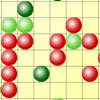Epidemic Modelling

Age 14 to 18 Challenge Level:

Use the computer to model an epidemic. Try out public health policies to control the spread of the epidemic, to minimise the number of sick days and deaths.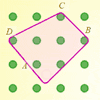Elastic Maths

Age 14 to 18

How do you write a computer program that creates the illusion of stretching elastic bands between pegs of a Geoboard? The answer contains some surprising mathematics.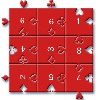Impuzzable

Age 16 to 18

This is about a fiendishly difficult jigsaw and how to solve it using a computer program.Shaping the Universe III - to Infinity and Beyond

Age 11 to 16

The third installment in our series on the shape of astronomical systems, this article explores galaxies and the universe beyond our solar system.Stonehenge

Age 16 to 18 Challenge Level:

Explain why, when moving heavy objects on rollers, the object moves twice as fast as the rollers. Try a similar experiment yourself.The Mean Game

Age 16 to 18

Edward Wallace based his A Level Statistics Project on The Mean Game. Each picks 2 numbers. The winner is the player who picks a number closest to the mean of all the numbers picked.Truth Tables and Electronic Circuits

Age 11 to 18

Investigate circuits and record your findings in this simple introduction to truth tables and logic.Lap Times

Age 14 to 16 Challenge Level:

Can you find the lap times of the two cyclists travelling at constant speeds?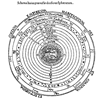Shaping the Universe II - the Solar System

Age 11 to 16

The second in a series of articles on visualising and modelling shapes in the history of astronomy.Shaping the Universe I - Planet Earth

Age 11 to 16

This article explores ths history of theories about the shape of our planet. It is the first in a series of articles looking at the significance of geometric shapes in the history of astronomy.Spot the Card

Age 14 to 16 Challenge Level:

It is possible to identify a particular card out of a pack of 15 with the use of some mathematical reasoning. What is this reasoning and can it be applied to other numbers of cards?Slippage

Age 14 to 16 Challenge Level:

A ladder 3m long rests against a wall with one end a short distance from its base. Between the wall and the base of a ladder is a garden storage box 1m tall and 1m high. What is the maximum distance. . . .Spinners

Age 16 to 18 Challenge Level:

How do scores on dice and factors of polynomials relate to each other?An Introduction to Computer Programming and Mathematics

Age 16 to 18

This article explains the concepts involved in scientific mathematical computing. It will be very useful and interesting to anyone interested in computer programming or mathematics.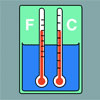Stemnrich - the Physical World

Age 11 to 16 Challenge Level:

PhysNRICH is the area of the StemNRICH site devoted to the mathematics underlying the study of physicsTwenty20

Age 7 to 16 Challenge Level:

Fancy a game of cricket? Here is a mathematical version you can play indoors without breaking any windows.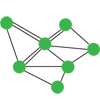Investigating Epidemics

Age 11 to 16 Challenge Level:

Simple models which help us to investigate how epidemics grow and die out.The Use of Mathematics in Computer Games

Age 16 to 18

An account of how mathematics is used in computer games including geometry, vectors, transformations, 3D graphics, graph theory and simulations.Witch's Hat

Age 11 to 16 Challenge Level:

What shapes should Elly cut out to make a witch's hat? How can she make a taller hat?Logic, Truth Tables and Switching Circuits Challenge

Age 11 to 18

Learn about the link between logical arguments and electronic circuits. Investigate the logical connectives by making and testing your own circuits and fill in the blanks in truth tables to record. . . .Time to Evolve 2

Age 16 to 18 Challenge Level:

How is the length of time between the birth of an animal and the birth of its great great ... great grandparent distributed?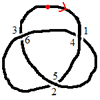Drawing Doodles and Naming Knots

Age 7 to 18

This article for students introduces the idea of naming knots using numbers. You'll need some paper and something to write with handy!What's a Knot?

Age 7 to 16 Challenge Level:

A brief video explaining the idea of a mathematical knot.Ball Bearings

Age 16 to 18 Challenge Level:

If a is the radius of the axle, b the radius of each ball-bearing, and c the radius of the hub, why does the number of ball bearings n determine the ratio c/a? Find a formula for c/a in terms of n.Chocolate 2010

Age 14 to 16 Challenge Level:

First of all, pick the number of times a week that you would like to eat chocolate. Multiply this number by 2...Problem Solving, Using and Applying and Functional Mathematics

Age 5 to 18 Challenge Level:

Problem solving is at the heart of the NRICH site. All the problems give learners opportunities to learn, develop or use mathematical concepts and skills. Read here for more information.Escalator

Age 14 to 16 Challenge Level:

At Holborn underground station there is a very long escalator. Two people are in a hurry and so climb the escalator as it is moving upwards, thus adding their speed to that of the moving steps. . . .Cushion Ball

Age 16 to 18 Challenge Level:

The shortest path between any two points on a snooker table is the straight line between them but what if the ball must bounce off one wall, or 2 walls, or 3 walls?
Concrete Calculation

Age 14 to 16 Challenge Level:

The builders have dug a hole in the ground to be filled with concrete for the foundations of our garage. How many cubic metres of ready-mix concrete should the builders order to fill this hole to. . . .Logic, Truth Tables and Switching Circuits

Age 11 to 18

Learn about the link between logical arguments and electronic circuits. Investigate the logical connectives by making and testing your own circuits and record your findings in truth tables.Population Dynamics - Part 2

Age 16 to 18 Challenge Level:

Second in our series of problems on population dynamics for advanced students.Rocking Chairs, Railway Games and Rayboxes

Age 5 to 18

In this article for teachers, Alan Parr looks at ways that mathematics teaching and learning can start from the useful and interesting things can we do with the subject, including. . . .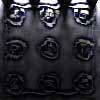Scratch Cards

Age 14 to 16 Challenge Level:

To win on a scratch card you have to uncover three numbers that add up to more than fifteen. What is the probability of winning a prize?Bird-brained

Age 16 to 18 Challenge Level:

How many eggs should a bird lay to maximise the number of chicks that will hatch? An introduction to optimisation.Pdf Stories

Age 16 to 18 Challenge Level:

Invent scenarios which would give rise to these probability density functions.Population Dynamics

Age 16 to 18 Challenge Level:

This problem opens a major sequence of activities on the mathematics of population dynamics for advanced students.Branching Processes and Extinction

Age 16 to 18 Challenge Level:

An advanced mathematical exploration supporting our series of articles on population dynamics for advanced students.Population Dynamics - Part 1

Age 16 to 18 Challenge Level:

First in our series of problems on population dynamics for advanced students.Population Dynamics - Part 3

Age 16 to 18 Challenge Level:

Third in our series of problems on population dynamics for advanced students.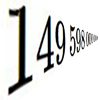Big and Small Numbers in Physics - Group Task

Age 16 to 18 Challenge Level:

Work in groups to try to create the best approximations to these physical quantities.Population Dynamics - Part 4

Age 16 to 18 Challenge Level:

Fourth in our series of problems on population dynamics for advanced students.Population Dynamics - Part 5

Age 16 to 18 Challenge Level:

Fifth in our series of problems on population dynamics for advanced students.Population Dynamics - Part 6

Age 16 to 18 Challenge Level:

Sixth in our series of problems on population dynamics for advanced students.Population Ecology Using Probability

Age 16 to 18 Challenge Level:

An advanced mathematical exploration supporting our series of articles on population dynamics for advanced students.Population Dynamics Collection

Age 16 to 18 Challenge Level:

This is our collection of tasks on the mathematical theme of 'Population Dynamics' for advanced students and those interested in mathematical modelling.Guessing the Graph

Age 14 to 16 Challenge Level:

Can you suggest a curve to fit some experimental data? Can you work out where the data might have come from?Overarch 2

Age 16 to 18 Challenge Level:

Bricks are 20cm long and 10cm high. How high could an arch be built without mortar on a flat horizontal surface, to overhang by 1 metre? How big an overhang is it possible to make like this?Designing Table Mats

Age 11 to 16 Challenge Level:

Formulate and investigate a simple mathematical model for the design of a table mat.The Not-so-simple Pendulum 1

Age 16 to 18 Challenge Level:

See how the motion of the simple pendulum is not-so-simple after all.Engnrich

Age 16 to 18 Challenge Level:

engNRICH is the area of the stemNRICH Advanced site devoted to the mathematics underlying the study of engineering